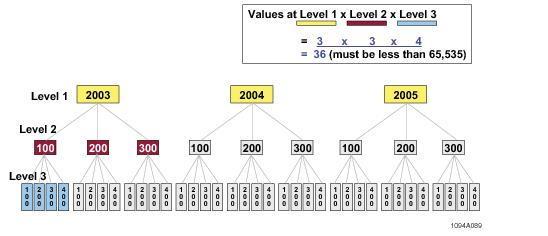# 15.00 - Combined Partitioning Expression for the Sales Table - Teradata Database

prodname
vrm_release
15.00
category
User Guide
featnum
B035-1094-015K

### Combined Partitioning Expression for the Sales Table

The corresponding combined partitioning expression is the following.

`     (RANGE_N(salesdate  BETWEEN DATE '2003-01-01' `
`                         AND     DATE '2005-12-31' `
`                         EACH INTERVAL '1' YEAR)-1)*12+`
`     (RANGE_N(storeid    BETWEEN 1 `
`                         AND   300 `
`                         EACH  100)-1)*4+`
`     (RANGE_N(productid  BETWEEN 1 `
`                         AND   400 `
`                         EACH  100)`

There are 3 row partitions for both the first level and second levels, and 4 row partitions for the third level. Therefore, the total number of combined partitions is the product of 3*3*4, or 36.

The following diagram indicates the logical hierarchy of the three row partitioning levels for sales (65,535 assumes 2-byte partitioning):